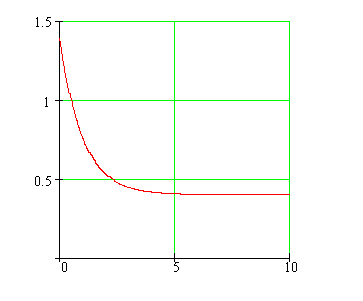Calculus on Demand at Dartmouth College Lecture 17 | Index | Lecture 19 Lecture 18Resources

Math 3 Course Syllabus
Practice Exams

Contents

In this lecture we continue with the discussion of separable differential equations. We also consider exponential growth and decay, important models in real-world applications.

Quick Question

Is the town population depicted in the following graph, growing, decreasing, or staying the same over the last ten years?Outline

Outlines for
Exponential Growth and Decay
Separable Differential Equations

Textbook

Exponential Growth and Decay
Separable Differential Equations